A spherometer is a device that does just as the name suggests: it measures (ometer) a sphere (sphere). But what exactly would you measure on a sphere? You could measure its diameter or the circumference of a great circle, the surface area or the radius. But all of these measurements will change proportionally to the radius R of the sphere. Any surface that is curved has a radius of curvature, the radius of the sphere that approximates the surface locally. Thus a spherometer can measure the radius of curvature of an item such as a lens and curved mirrors that are spherical in shape.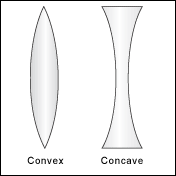The first known spherometer was invented by French optician Robert-Aglaé Cauchoix in 1810. They were manufactured starting in the nineteenth century primarily for the use of opticians in grinding lenses and astronomers in grinding lenses and curved mirrors. Though there are several shapes of lenses, most lenses are spherical in that the curve of the lens is a portion of a sphere. See the examples of a bi-convex and a bi-concave lens to the right.

Engineers also used spherometers to measure curvature as well the thickness of objects such as metal plates. Machinists use them to measure the depth of depressions or raised areas on surfaces. This allows for weak points in items such as oil well piping to be discovered before being shipped to a well site. They were also used in the physics classroom to conduct experiments. One such common experiment would be to produce Newton’s ring. By refracting light through a curved and a flat medium (a lens and the flat), an interference pattern of concentric dark and light rings appears.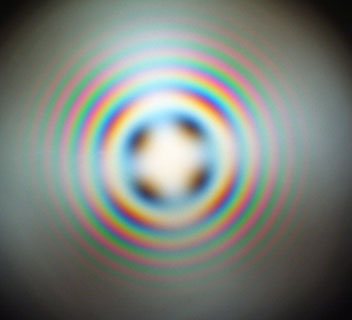Newton's Ring

Though amateur astronomers still sometimes grind their own lenses, most lens and mirror production is now largely automated. Spherometers are still made, many look much like to older ones or have analog gages instead of scales. Several companies produce electronic versions that yet still rely on the same underlying theory.

Most spherometers were small devices, usually ranging from four or five centimeters to less than twenty centimeters across and were predominantly used by lens makers to precisely measure the curvature of a lens. Given the delicate nature of these devices, they are easily bent, so they often come with a glass plate, called a flat, that can be used to zero the micrometer. Spherometers have three legs that form an equilateral triangle. Recall from geometry that three points determine a plane, so the three legs will always touch the glass. But you also know from experience that four points do not, think of a wobbly café table. A fourth leg or point is located at the center the three legs. This central point is attached to a micrometer screw that can be raised or lowered. Micrometers are devices such as calipers that translate very small measurements into larger movements of the device to allow precise measurements. One complete turn of the central screw of the spherometer raises or lowers the center point a set distance which is determined by the size of the thread of the screw and the radius of the disc. Each rotation of the screw raises the disc one unit on the vertical scale. To make measurements to two decimal places, the horizontal disc of the spherometer is often marked to give readings to hundredths of a rotation. The distance the central point is above or below the plane made by the three legs is then multiplied by the length associated with one turn of the micrometer. In this way a spherometer can measure both a positively or negatively curved surface to high accuracy.

The mathematics behind the device uses only high school trigonometry and the mathematics of right triangles. If the distance between any two of the three legs is denoted by a, then the distance from any of the three legs to the central point is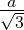.

The micrometer measures the distance above or below the plane of the three legs. In optics, the distance h is called the sagitta, referred to using the letter h or s, for the depth of glass removed from a lens. Using h, the standard equation for the curvature of a sphere is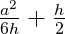.

See the figure below for a view of the lens and spherometer from the side (with only two of the three legs shown).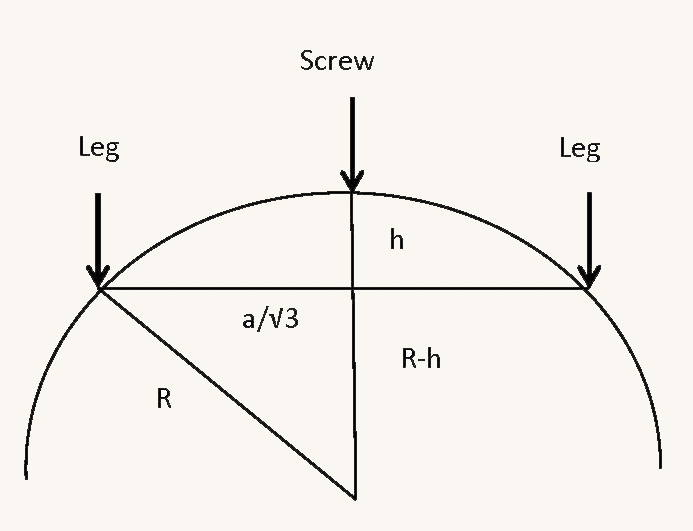## References

Stargazerslounge.com

Mills, A. “The Spherometer”, eRittnehouse, Vol 24, 2012/2013

Warner, D.J., “Spherometer”, Instruments of Science: An Historical Encyclopedia, 1998, pp.569-570